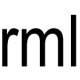# Triangle Identification – C++ solution and test code

Here is another interview coding question that I have heard of.

You have to write a function to identify the triangle type. You have to return a number between 1 or 4 to identify either a valid triangle type or an error.

The solution is very simple requiring only one condensed line of code to identify each triangle type. Two lines of code identify error cases. One of the error cases identifies input with 0 or negative lengths. The other error case identifies line lengths that don’t make a triangle or which make a segment (thanks to Yabba for pointing out these error cases).

As an exercise you could try to extend the solution to identify right-angled triangles as well.

```const int SCALENE = 1;
const int ISOSCELES = 2;
const int EQUILATERAL = 3;
const int ERROR = 4;

int TriangleType(int x, int y, int z)
{
if ((x + y <= z) || (x + z <= y) || (z + y <= x)) return ERROR;
if (x<=0 || y<=0 || z<=0) return ERROR;
if (x == y && y == z) return EQUILATERAL;
if (x == y || y == z || z == x) return ISOSCELES;
return SCALENE;
}
```

The more interesting code comes from testing the function. To test the function I pass in a series of triangle side lengths. I also pass in the expected result. If the actual result matches the expected result then the test passes.

The test data is written in a way that allows each test to be defined in a single line of code which makes it very easy to extend.

This easy extendibility was fortunate as my original version of this solution missed out the cases where the line lengths don’t make a triangle (e.g. 1, 1, 5), and where the line lengths make a segment (e.g. 2, 2, 4).

```void TriangleTest()
{
const int testDataSize = 14;

int testData[testDataSize] = {
{9, 9, 9, EQUILATERAL},
{4, 5, 5, ISOSCELES},
{5, 4, 5, ISOSCELES},
{5, 3, 3, ISOSCELES},
{0, 0, 0, ERROR}, // 0 number checks
{0, 1, 2, ERROR},
{1, 0, 2, ERROR},
{1, 2, 0, ERROR},
{-1, -1, -1, ERROR}, // negative number checks
{-1, 1, 2, ERROR},
{1, -1, 2, ERROR},
{1, 2, -1, ERROR},
{2, 2, 4, ERROR}, // line segment not a triangle
{1, 1, 5, ERROR} // not a triangle
};

for (int i=0; i<testDataSize; ++i)
{
int result = TriangleType(
testData[i], testData[i], testData[i]);
if (result == testData[i])
{
cout << " Pass: triangleType("
<< testData[i] << ", "
<< testData[i] << ", "
<< testData[i] << ")==" << result << endl;
}
else
{
cout << "!Fail: triangleType("
<< testData[i] << ", "
<< testData[i] << ", "
<< testData[i] << ")==" << result
<< " Expected: " << testData[i] << endl;
}
}
}
```

### 2 Comments on “Triangle Identification – C++ solution and test code”

1.Yabba says:

{2, 2, 4, ISOSCELES} – this is segment not a triangle
{1, 1, 5, ERROR} – not ISOSCELES

2.admin says:

Thanks Yabba! I’ve now updated the post with your new tests, and with code to identify the errors.